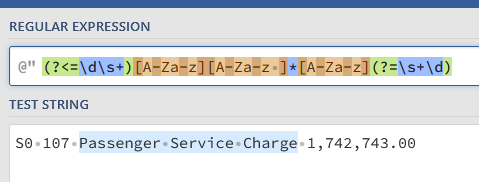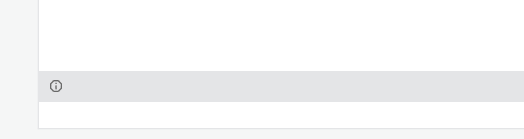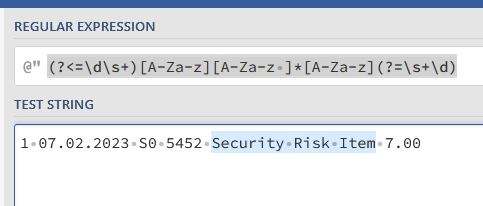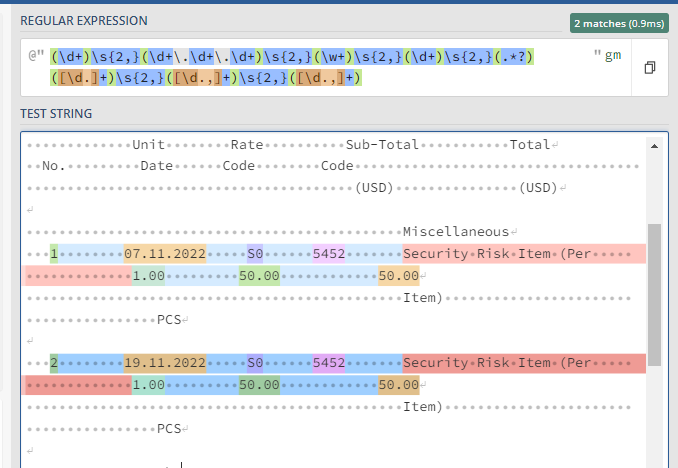# Regex to get str values between numbers

S0 107 Passenger Service Charge 1,742,743.00

hi, how can i get the ‘Passenger Service Charge’ string? The numbers before and after the string are not fixed. The ‘Passenger Service Charge’ string also is not fixed.

I just want to get the value(string) in between the left and right numbers.

HI @syezids

Checkout this expression

``````System.Text.RegularExpressions.Regex.Match(InputString,"(?<=\d\s)\D+(?=\s\d)").Tostring
``````

Regards
Sudharsan

Hi,

Can you try the following pattern?

``````(?<=\d\s+)[A-Za-z][A-Za-z ]*[A-Za-z](?=\s+\d)
``````Regards,

hi, i tested with this and success for most of strings, but failed on this one:
1 07.02.2023 S0 5452 Security Risk Item 7.00

Any idea why? is it due to extra number columns before?

Have you tested this ?@syezidsRegards
Sudharsan

hi, ive tested with yours
System.Text.RegularExpressions.Regex.Match(outreg,“(?<=\d\s)\D+(?=\s\d)”).Tostring

but it didnt produced any output, just empty stringHi @syezids ,

Check this below code,
System.Text.RegularExpressions.Regex.Match(outreg,“(?<=\d\s)\D+(?=\s\d)”,System.Text.RegularExpressions.RegexOptions.Multiline).Value

i have this error:

Assign: Expression Activity type ‘VisualBasicValue`1’ requires compilation in order to run. Please ensure that the workflow has been compiled.

Try this @syezids

``````System.Text.RegularExpressions.Regex.Match(outreg,“(?<=\d\s)[A-Z\sa-z]+(?=\s\d)”).Tostring
``````

Regards
Sudharsan

Hi @syezids ,

Can you check below code and let us know,
`System.Text.RegularExpressions.Regex.Match(outreg,"(?<=\d\s)\D+(?=\s\d)",System.Text.RegularExpressions.RegexOptions.Multiline).Value`

Or

`System.Text.RegularExpressions.Regex.Match(outreg,"(?<=\d\s+)[A-Za-z][A-Za-z ]*[A-Za-z](?=\s+\d)",System.Text.RegularExpressions.RegexOptions.Multiline).Value`

Hope this may help younvm i solved that error due to incorrect quotation used, however still not getting result, still empty string

Hi,

The above pattern seems to work as the following.Is this wrong?

Regards,

i have tested all the suggested solution, but still finds that bot is logging empty string instead of the middle variables.
Other row does not matter as the variable needed is the one underlined in red.

Can you send this text here? @syezids

invoice 1 with format.txt (2.1 KB)

Try this @syezids

``````System.Text.RegularExpressions.Regex.Matches(outreg,“(?<=\d)\s+\D+(?=\())”)
``````

You will get the data from the index starting from 1 ,2,…till last

``````System.Text.RegularExpressions.Regex.Matches(outreg,“(?<=\d)\s+\D+(?=\())”)(1).Tostring.Trim
``````
``````System.Text.RegularExpressions.Regex.Matches(outreg,“(?<=\d)\s+\D+(?=\())”)(2).Tostring.Trim
``````

And the index is dynamic but in your case it should be starts from 1

Regards
Sudharsan

Hi @syezids ,

I believe, you would require to get the data in the form of a Table, If that is the case you could check with the below Expression :

``````(\d+)\s{2,}(\d+\.\d+\.\d+)\s{2,}(\w+)\s{2,}(\d+)\s{2,}(.*?)([\d.]+)\s{2,}([\d.,]+)\s{2,}([\d.,]+)
``````Also, Follow the Steps mentioned in the below post, but suit it to your requirements, Like change the regex expression to the above, add the necessary columns to the `Build Datatable` Activity as per your need :

Before using or following the approach confirm on whether the Table/Row by Row extraction is really what you need.

1 Like

thanks a lot for your fast input, i have managed to combine all of your suggested solution accordingly to work with my requirements and now is working fine. cheers.

1 Like